# Rotational diffusion

Rotational diffusion is a process by which the equilibrium statistical distribution of the overall orientation of particles or molecules is maintained or restored. Rotational diffusion is the counterpart of translational diffusion, which maintains or restores the equilibrium statistical distribution of particles' position in space.

If a molecule has an orientation along a unit vector n, and f(θ, φ, t) represents the probability density distribution for the orientation of n at time t (with the usual meaning of the θ and φ angles in spherical coordinates). The rotational diffusion equation is: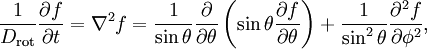$\frac{1}{D_{\mathrm{rot}}} \frac{\partial f}{\partial t} = \nabla^{2} f = \frac{1}{\sin\theta} \frac{\partial}{\partial \theta}\left( \sin\theta \frac{\partial f}{\partial \theta} \right) + \frac{1}{\sin^{2} \theta} \frac{\partial^{2} f}{\partial \phi^{2}},$

with the rotational diffusion coefficient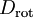$D_\mathrm{rot}$, which has units of inverse time.

This partial differential equation may be solved by expanding f(θ, φ, t) in spherical harmonics, for which the mathematical identity holds: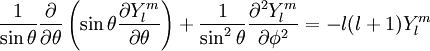$\frac{1}{\sin\theta} \frac{\partial}{\partial \theta}\left( \sin\theta \frac{\partial Y^{m}_{l}}{\partial \theta} \right) + \frac{1}{\sin^{2} \theta} \frac{\partial^{2} Y^{m}_{l}}{\partial \phi^{2}} = -l(l+1) Y^{m}_{l}$

Thus, the solution of this partial differential equation may be written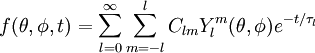$f(\theta, \phi, t) = \sum_{l=0}^{\infty} \sum_{m=-l}^{l} C_{lm} Y^{m}_{l}(\theta, \phi) e^{-t/\tau_{l}}$

where Clm are constants determined by the initial distribution. The most relevant result is the one for relaxation times: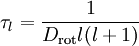$\tau_{l} = \frac{1}{D_{\mathrm{rot}}l(l+1)}$